# In triangle

In triangle ABC, the magnitude of the internal angle gamma is equal to one-third of the angle alpha. The size of the angle beta is 80 degrees larger than the size of the gamma angle. Calculate the magnitudes of the interior angles of the triangle ABC.

A =  60
B =  100
C =  20

### Step-by-step explanation:

A+B+C = 180
C = A/3
B = 80+C

A+B+C = 180
A-3C = 0
B-C = 80

A = 60
B = 100
C = 20

Our linear equations calculator calculates it.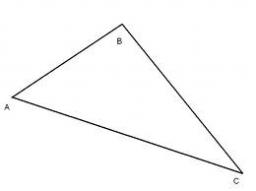Did you find an error or inaccuracy? Feel free to write us. Thank you!Tips to related online calculators
Do you have a linear equation or system of equations and looking for its solution? Or do you have a quadratic equation?

#### You need to know the following knowledge to solve this word math problem:

We encourage you to watch this tutorial video on this math problem:

## Related math problems and questions:

• Alfa beta gamaThe triangle's an interior angle beta is 10 degrees greater than the angle alpha and gamma angle is three times larger than the beta. Determine the size of the interior angles.
• Alfa, beta, gamaIn the triangle ABC is the size of the internal angle BETA 8 degrees larger than the size of the internal angle ALFA and size of the internal angle GAMA is twice the size of the angle BETA. Determine the size of the interior angles of the triangle ABC.
• Angles of the triangleABC is a triangle. The size of the angles alpha, beta are in a ratio 4: 7. The angle gamma is greater than the angle alpha by a quarter of a straight angle. Determine angles of the triangle ABC.
• Angles of a triangleIn triangle ABC, the angle beta is 15° greater than the angle alpha. The remaining angle is 30° greater than the sum of the angles alpha and beta. Calculate the angles of a triangle.
• Angles in triangle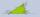Calculate the alpha angle in the triangle if beta is 61 degrees and 98 gamma degrees.
• Internal anglesOne internal angle of the triangle JAR is 25 degrees. The difference is the size of the two others is 15°. Identify the size of these angles.
• Internal and external anglesCalculate the remaining internal and external angles of a triangle, if you know the internal angle γ (gamma) = 34 degrees and one external angle is 78 degrees and 40 '. Determine what kind of triangle it is from the size of its angles.
• Triangle anglesIn a triangle, ABC, the interior angle at vertex C is twice the internal angle at point A. The outer angle at point B measured 117 degrees. How big is the external angle at vertex A?
• Internal angles IST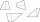Determine internal angles of isosceles trapezium ABCD /a, c are the bases/ and if: alpha:gamma = 1:3
• Angles ratioIn a triangle ABC, is true relationship c is less than b, and b is less than a. Internal angles of the triangle are in the ratio 5:4:9. The size of the internal angle beta is:
• The second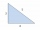The second angle of a triangle is the same size as the first angle. The third angle is 12 degrees larger than the first angle. How large are the angles?
• Internal anglesFind the internal angles of the triangle ABC if the angle at the vertex C is twice the angle at the B and the angle at the vertex B is 4 degrees smaller than the angle at vertex A.
• Centre of the hypotenuseFor the interior angles of the triangle ABC, alpha beta and gamma are in a ratio of 1: 2: 3. The longest side of the AB triangle is 30 cm long. Calculate the perimeter of the triangle CBS if S is the center of the side AB.
• Angles ratioThe internal angles of a triangle are in ratio 1:4:5. What kind of triangle is it? (solve interior angles and write down and discuss)
• Supplementary angles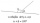One of the supplementary angles is larger by 33° than the second one. Calculate the angles size.
• Angles in ratioThe size of the angles of the triangle are in ratio x: y = 7: 5 and the angle z is 42° lower than the angle y. Find size of the angles x, y, z.
• Inscribed circle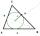Calculate the magnitude of the BAC angle in the triangle ABC if you know that it is 3 times less than the angle BOC, where O is the center of the circle inscribed in the triangle ABC.